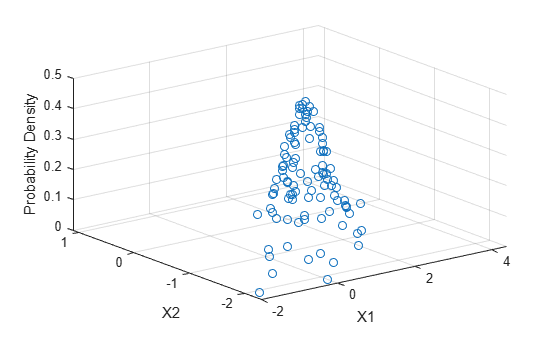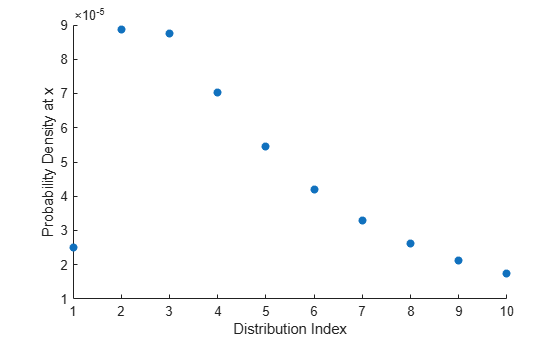# mvnpdf

Multivariate normal probability density function

## Syntax

``y = mvnpdf(X)``
``y = mvnpdf(X,mu)``
``y = mvnpdf(X,mu,Sigma)``

## Description

example

````y = mvnpdf(X)` returns an n-by-`1` vector `y` containing the probability density function (pdf) values for the d-dimensional multivariate normal distribution with zero mean and identity covariance matrix, evaluated at each row of the n-by-d matrix `X`. For more information, see Multivariate Normal Distribution.```

example

````y = mvnpdf(X,mu)` returns pdf values of points in `X`, where `mu` determines the mean of each associated multivariate normal distribution.```

example

````y = mvnpdf(X,mu,Sigma)` returns pdf values of points in `X`, where `Sigma` determines the covariance of each associated multivariate normal distribution.Specify `[]` for `mu` to use its default value of zero when you want to specify only `Sigma`. ```

## Examples

collapse all

Evaluate the pdf of a standard five-dimensional normal distribution at a set of random points.

Randomly sample eight points from the standard five-dimensional normal distribution.

```mu = zeros(1,5); Sigma = eye(5); rng('default') % For reproducibility X = mvnrnd(mu,Sigma,8)```
```X = 8×5 0.5377 3.5784 -0.1241 0.4889 -1.0689 1.8339 2.7694 1.4897 1.0347 -0.8095 -2.2588 -1.3499 1.4090 0.7269 -2.9443 0.8622 3.0349 1.4172 -0.3034 1.4384 0.3188 0.7254 0.6715 0.2939 0.3252 -1.3077 -0.0631 -1.2075 -0.7873 -0.7549 -0.4336 0.7147 0.7172 0.8884 1.3703 0.3426 -0.2050 1.6302 -1.1471 -1.7115 ```

Evaluate the pdf of the distribution at the points in `X`.

`y = mvnpdf(X)`
```y = 8×1 0.0000 0.0000 0.0000 0.0000 0.0054 0.0011 0.0015 0.0003 ```

Find the point in `X` with the greatest pdf value.

`[maxpdf,idx] = max(y)`
```maxpdf = 0.0054 ```
```idx = 5 ```
`maxPoint = X(idx,:)`
```maxPoint = 1×5 0.3188 0.7254 0.6715 0.2939 0.3252 ```

The fifth point in `X` has a greater pdf value than any of the other randomly selected points.

Create six three-dimensional normal distributions, each with a distinct mean. Evaluate the pdf of each distribution at a different random point.

Specify the means `mu` and covariances `Sigma` of the distributions. Each distribution has the same covariance matrix—the identity matrix.

```firstDim = (1:6)'; mu = repmat(firstDim,1,3)```
```mu = 6×3 1 1 1 2 2 2 3 3 3 4 4 4 5 5 5 6 6 6 ```
`Sigma = eye(3)`
```Sigma = 3×3 1 0 0 0 1 0 0 0 1 ```

Randomly sample once from each of the six distributions.

```rng('default') % For reproducibility X = mvnrnd(mu,Sigma)```
```X = 6×3 1.5377 0.5664 1.7254 3.8339 2.3426 1.9369 0.7412 6.5784 3.7147 4.8622 6.7694 3.7950 5.3188 3.6501 4.8759 4.6923 9.0349 7.4897 ```

Evaluate the pdfs of the distributions at the points in `X`. The pdf of the first distribution is evaluated at the point `X(1,:)`, the pdf of the second distribution is evaluated at the point `X(2,:)`, and so on.

`y = mvnpdf(X,mu)`
```y = 6×1 0.0384 0.0111 0.0000 0.0009 0.0241 0.0001 ```

Evaluate the pdf of a two-dimensional normal distribution at a set of given points.

Specify the mean `mu` and covariance `Sigma` of the distribution.

```mu = [1 -1]; Sigma = [0.9 0.4; 0.4 0.3];```

Randomly sample from the distribution 100 times. Specify `X` as the matrix of sampled points.

```rng('default') % For reproducibility X = mvnrnd(mu,Sigma,100);```

Evaluate the pdf of the distribution at the points in `X`.

`y = mvnpdf(X,mu,Sigma);`

Plot the probability density values.

```scatter3(X(:,1),X(:,2),y) xlabel('X1') ylabel('X2') zlabel('Probability Density')```Create ten different five-dimensional normal distributions, and compare the values of their pdfs at a specified point.

Set the dimensions `n` and `d` equal to 10 and 5, respectively.

```n = 10; d = 5;```

Specify the means `mu` and the covariances `Sigma` of the multivariate normal distributions. Let all the distributions have the same mean vector, but vary the covariance matrices.

`mu = ones(1,d)`
```mu = 1×5 1 1 1 1 1 ```
```mat = eye(d); nMat = repmat(mat,1,1,n); var = reshape(1:n,1,1,n); Sigma = nMat.*var;```

Display the first two covariance matrices in `Sigma`.

`Sigma(:,:,1:2)`
```ans = ans(:,:,1) = 1 0 0 0 0 0 1 0 0 0 0 0 1 0 0 0 0 0 1 0 0 0 0 0 1 ans(:,:,2) = 2 0 0 0 0 0 2 0 0 0 0 0 2 0 0 0 0 0 2 0 0 0 0 0 2 ```

Set `x` to be a random point in five-dimensional space.

```rng('default') % For reproducibility x = normrnd(0,1,1,5)```
```x = 1×5 0.5377 1.8339 -2.2588 0.8622 0.3188 ```

Evaluate the pdf at `x` for each of the ten distributions.

`y = mvnpdf(x,mu,Sigma)`
```y = 10×1 10-4 × 0.2490 0.8867 0.8755 0.7035 0.5438 0.4211 0.3305 0.2635 0.2134 0.1753 ```

Plot the results.

```scatter(1:n,y,'filled') xlabel('Distribution Index') ylabel('Probability Density at x')```## Input Arguments

collapse all

Evaluation points, specified as a `1`-by-d numeric vector or an n-by-d numeric matrix, where n is a positive scalar integer and d is the dimension of a single multivariate normal distribution. The rows of `X` correspond to observations (or points), and the columns correspond to variables (or coordinates).

If `X` is a vector, then `mvnpdf` replicates it to match the leading dimension of `mu` or the trailing dimension of `Sigma`.

Data Types: `single` | `double`

Means of multivariate normal distributions, specified as a `1`-by-d numeric vector or an n-by-d numeric matrix.

• If `mu` is a vector, then `mvnpdf` replicates the vector to match the trailing dimension of `Sigma`.

• If `mu` is a matrix, then each row of `mu` is the mean vector of a single multivariate normal distribution.

Data Types: `single` | `double`

Covariances of multivariate normal distributions, specified as a d-by-d symmetric, positive definite matrix or a d-by-d-by-n numeric array.

• If `Sigma` is a matrix, then `mvnpdf` replicates the matrix to match the number of rows in `mu`.

• If `Sigma` is an array, then each page of `Sigma`, `Sigma(:,:,i)`, is the covariance matrix of a single multivariate normal distribution and, therefore, is a symmetric, positive definite matrix.

If the covariance matrices are diagonal, containing variances along the diagonal and zero covariances off it, then you can also specify `Sigma` as a `1`-by-d vector or a `1`-by-d-by-n array containing just the diagonal entries.

Data Types: `single` | `double`

## Output Arguments

collapse all

pdf values, returned as an n-by-`1` numeric vector, where n is one of the following:

• Number of rows in `X` if `X` is a matrix

• Number of times `X` is replicated if `X` is a vector

If `X` is a matrix, `mu` is a matrix, and `Sigma` is an array, then `mvnpdf` computes `y(i)` using `X(i,:)`, `mu(i,:)`, and `Sigma(:,:,i)`.

Data Types: `double`

collapse all

### Multivariate Normal Distribution

The multivariate normal distribution is a generalization of the univariate normal distribution to two or more variables. It has two parameters, a mean vector μ and a covariance matrix Σ, that are analogous to the mean and variance parameters of a univariate normal distribution. The diagonal elements of Σ contain the variances for each variable, and the off-diagonal elements of Σ contain the covariances between variables.

The probability density function (pdf) of the d-dimensional multivariate normal distribution is

where x and μ are 1-by-d vectors and Σ is a d-by-d symmetric, positive definite matrix. Only `mvnrnd` allows positive semi-definite Σ matrices, which can be singular. The pdf cannot have the same form when Σ is singular.

The multivariate normal cumulative distribution function (cdf) evaluated at x is the probability that a random vector v, distributed as multivariate normal, lies within the semi-infinite rectangle with upper limits defined by x:

`$\mathrm{Pr}\left\{v\left(1\right)\le x\left(1\right),v\left(2\right)\le x\left(2\right),...,v\left(d\right)\le x\left(d\right)\right\}.$`

Although the multivariate normal cdf does not have a closed form, `mvncdf` can compute cdf values numerically.

## Tips

• In the one-dimensional case, `Sigma` is the variance, not the standard deviation. For example, `mvnpdf(1,0,4)` is the same as `normpdf(1,0,2)`, where `4` is the variance and `2` is the standard deviation.

 Kotz, S., N. Balakrishnan, and N. L. Johnson. Continuous Multivariate Distributions: Volume 1: Models and Applications. 2nd ed. New York: John Wiley & Sons, Inc., 2000.